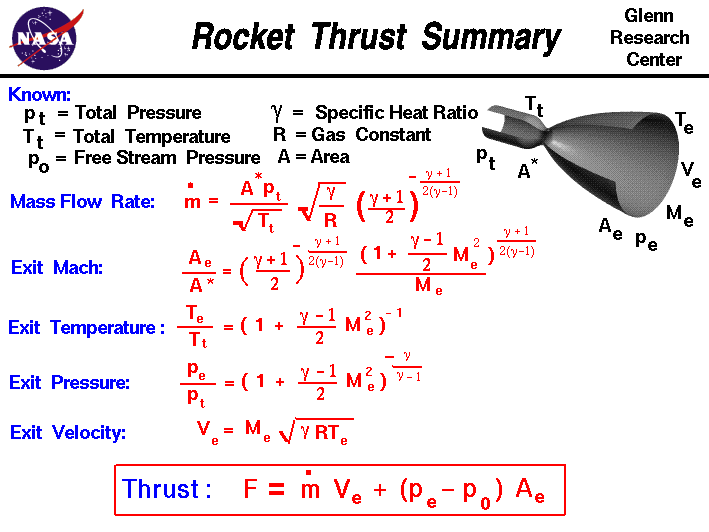+ Text Only Site
+ Non-Flash Version
+ Contact GlennOn this slide, we have collected all of the equations necessary to calculate the thrust of a rocket engine. In a rocket engine, stored fuel and stored oxidizer are ignited in a combustion chamber. The combustion produces great amounts of exhaust gas at high temperature and pressure. The hot exhaust is passed through a nozzle which accelerates the flow. Thrust is produced according to Newton's third law of motion.

The amount of thrust produced by the rocket depends on the mass flow rate through the engine, the exit velocity of the exhaust, and the pressure at the nozzle exit. All of these variables depend on the design of the nozzle. The smallest cross-sectional area of the nozzle is called the throat of the nozzle. The hot exhaust flow is choked at the throat, which means that the Mach number is equal to 1.0 in the throat and the mass flow rate m dot is determined by the throat area.

mdot = (A* * pt/sqrt[Tt]) * sqrt(gam/R) * [(gam + 1)/2]^-[(gam + 1)/(gam - 1)/2]

where A* is the area of the throat, pt is the total pressure in the combustion chamber, Tt is the total temperature in the combustion chamber, gam is the ratio of specific heats of the exhaust, and R is the gas constant.

The area ratio from the throat to the exit Ae sets the exit Mach number:

A/A* = {[(gam+1)/2]^-[(gam+1)/(gam-1)/2]} / Me * [1 + Me^2 * (gam-1)/2]^[(gam+1)/(gam-1)/2]

Solving for the exit Mach number when we know the exit area ratio is quite difficult. But, we can use a computer program to iteratively solve the equation. Here's a JavaScript program that solves for the Mach number when you specify the area ratio:

# Isentropic Flow Calculator

Input
Output
Mach
Mach Angle
P-M Ang
p/pt
T/Tt
rho/rhot
q/p
A/A*
Wcor/A

By default, the program Input Variable is the Mach number of the flow. Since the area ratio depends only on the Mach number and ratio of specific heats, the program can calculate the value of the area ratio and display the results on the right side of the output variables. You can also have the program solve for the Mach number that produces a desired value of area ratio. Using the choice button labeled Input Variable, select "Area Ratio - A/A*". Next to the selection, you then type in a value for A/A*. When you hit the red COMPUTE button, the output values change. The area ratio is double valued; for the same area ratio, there is a subsonic and a supersonic solution. The choice button at the right top selects the solution that is presented.

If you are an experienced user of this calculator, you can use a sleek version of the program which loads faster on your computer and does not include these instructions. You can also download your own copy of the program to run off-line by clicking on this button:

We can determine the exit pressure pe and exit temperature Te from the isentropic relations at the nozzle exit:

pe / pt = [1 + Me^2 * (gam-1)/2]^-[gam/(gam-1)]

Te / Tt = [1 + Me^2 * (gam-1)/2]^-1

Knowing Te we can use the equation for the speed of sound and the definition of the Mach number to calculate the exit velocity Ve:

Ve = Me * sqrt (gam * R * Te)

We now have all the information necessary to determine the thrust of a rocket. The exit pressure is only equal to free stream pressure at some design condition. We must, therefore, use the longer version of the generalized thrust equation to describe the thrust of the system. If the free stream pressure is given by p0, the rocket thrust equation is given by:

F = m dot * Ve + (pe - p0) * Ae

You can explore the design and operation of a rocket nozzle with our interactive nozzle simulator program which runs on your browser.

The thrust equation shown above works for both liquid rocket and solid rocket engines. There is also an efficiency parameter called the specific impulse which works for both types of rockets and greatly simplifies the performance analysis for rockets.

Activities:

Guided Tours+ Inspector General Hotline + Equal Employment Opportunity Data Posted Pursuant to the No Fear Act + Budgets, Strategic Plans and Accountability Reports + Freedom of Information Act + The President's Management Agenda + NASA Privacy Statement, Disclaimer, and Accessibility CertificationEditor: Nancy Hall NASA Official: Nancy Hall Last Updated: May 13 2021 + Contact Glenn